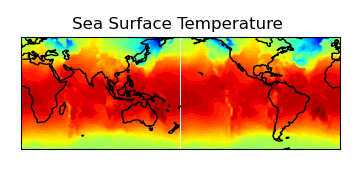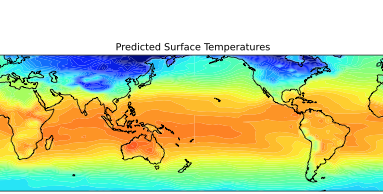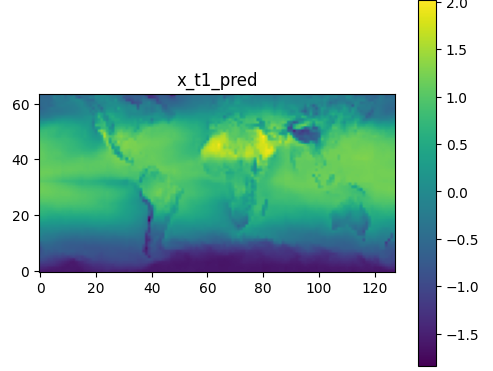# Fourcastnet inference duplicate output

I have successfully trained a model using Fourcastnet. I have been using the inferencer.py code to capture the true and recursive_pred output of a trained model. The input is on128x64 grid.

When I plot the output of both the true and predicted data I seem to have two 64x64 versions of the full grid in the 128x64 output data. I am expecting a 128x64 output grid matching the input?

I have verified that the input data is the correct 128x64 field without duplicates.

Is this an issue with a setting or a bug?

The figure shows the two 64x64 grids in the 128x64 output array produced by the model. The lines at 180 degrees are an artefact of the plotting (no wrap points included).Here is the input data:-This is the code that I use to extract the data:-

surft_p[nsave, tstep,:,:] = pred_recursive[0,:,:].cpu().detach().numpy()
surft_t[nsave, tstep,:,:] = true[0,:,:].cpu().detach().numpy()

That is odd, was the model trained on `128 x 64` data? Also did you set the `img_shape` to the correct size for the tiling? Looking at your outputs it looks like the output is shifted (I can see the Andes temp in the middle of the pacific). Could this be a potential clue?

I have now confirmed that the dataloader call in inferencer.py:-

for tstep, (invar, true_outvar, _) in enumerate(dataloader):

returns Invar as a 128x64 field. true_outvar is two 64x64 fields and the call to model returns pred_outvar_recursive as two 64x64 fields. So the Dataloader is the source of the error.

Yes the model was trained on 128x64 data correctly. The plot labelled sea surface temperature (above) is the input data on a 128x64 grid taken from an input file.

Note also that both the predicted and true data fields are both on a 64x64 grid. This will mean that you will still get credible estimates of RMSE and ACC using inferencer.py so you would only spot this problem if you plot the data as I have done.

Here is an example of one half of the 128x64 output field ie 64x64. You can see that this is can be correctly mapped to the full world map assuming that there are only 64 longitude point not 128. It is odd that it is only happening with the longitude dimension. Note also that the two 64x64 grids for both the predicted and true fields are different ie not simple repeats.Here is the output from inferencer.py

invar = {‘x_t0’: tensor([[[[-1.3271, -1.2201, -1.1344, …, -1.2121, -1.2679, -1.5170],
[-1.3434, -1.2300, -1.1234, …, -1.1773, -1.3166, -1.5388],
[-1.3759, -1.2575, -1.1204, …, -1.2088, -1.3996, -1.5502],
…,
[-1.4543, -1.2096, -1.0638, …, -1.3316, -1.4448, -3.2561],
[-1.4061, -1.2203, -1.1135, …, -1.2970, -1.3545, -2.7634],
[-1.3567, -1.2162, -1.0895, …, -1.2672, -1.2819, -1.5542]]]],
device=‘cuda:0’)} [128, 64]
pred_outvar_recursive torch.Size([1, 128, 64])

here is a plot from the validator output showing the expected output: -Thanks for looking into this. I’ll make sure we have a look at the dataloader/dataset from our side to figure out what the issue is.

So to clarify the input tensor is `128x64` and the true_outvar tensor is `2x64x64`? But the model correctly gives pred_outvar_recursive to be `128x64`? Want to make sure I know exactly what tensors are the correct and incorrect shape to try to replicate. Thanks!

I have now solved the problem. It seems to be a PyTorch error as It relates to converting a PyTorch tensor to a numpy array. The conversion to a numpy array does not check the shape and throw an error when [128x64] tensor was written to an [64x128] numpy array, so this error remained invisible:-

surft_p = np.transpose(pred_recursive[0,:,:].cpu().detach().numpy())

Note that the root cause of this problem is that the original ECMWF data, written in netcdf format, follows the accepted standard meteorological community standards and is written as a […,latitude, longitude] data set. The HDF5 files used by Fourcastnet have reversed this and use a […,longitude, latitude] format, not consistent with the accepted meteorological format. I recommend that Fourcastnet use the netcdf file format as used by ECMWF and that the data be formatted […,latitude, longitude]. Here is an example field from an ECMWF data file: -

short sst(time, latitude, longitude) ;
sst:scale_factor = 0.000586115577027986 ;